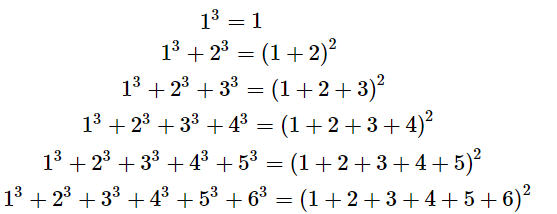# Observe the following pattern:`
Question:

Observe the following pattern:
13 = 1
13 + 23 = (1 + 2)2
13 + 23 + 33 = (1 + 2 + 3)2
Write the next three rows and calculate the value of 13 + 2+ 33 + ... + 9+ 103 by the above pattern.

Solution:

Extend the pattern as follows:Now, from the above pattern, the required value is given by:

$1^{3}+2^{3}+3^{3}+4^{3}+5^{3}+6^{3}+7^{3}+8^{3}+9^{3}+10^{3}=(1+2+3+4+5+6+7+8+9+10)^{2}=55^{2}$

= 3025

Thus, the required value is 3025 .